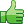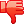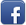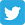# POST into Array problem

Posted on 16th Feb 2014 07:03 pm by admin

Hi Chaps,

I have a repeat region, displaying rows of data: jobid, fromtable, translatorcharge
In each row there is an input field to enter a cost for each job 'charge'.
//INPUT - TRANSLATOR CHARGE
Code: (text) <input type="text" name="translatorcharge" id="count" class="price" value="<?php echo \$row_rsInvPending['jobtranslatorcharge']; ?>"/>
I have a hidden input that collects the information for each row, before sending all the information to a script page:
//HIDDEN INPUT - INFO COLLECTOR
Code: (text) <?php
\$table_name = \$row_rsInvPending['fromtable'];
\$item_id = \$row_rsInvPending['jobid'];
?>
<input type="hidden" name="jobinvsent[]" value="<?php echo \$table_name; ?>:<?php echo \$item_id; ?>:<?php \$_POST['translatorcharge'] ?>" />
The script page then updates the translatorcharge column in the relevant table:
//SCRIPT - TO UPDATE DATABASE
Code: (text) \$allowed_tables = Array('tbl_jobs','tbl_jobtransline','tbl_jobxml'); // to prevent SQL injection
foreach(\$_POST['jobinvsent'] as \$var) {
\$arr = explode(':', \$var);
if(in_array(\$arr, \$allowed_tables)) {
\$table = \$arr;
\$rowid = \$arr;
\$transcharge = \$_POST['translatorcharge'];
if(is_numeric(\$rowid)) {
// run your SQL query here to update \$table where row matches \$rowid
\$mess = \$ref = \$_SERVER['HTTP_REFERER']; header( 'refresh: 0; url='.\$ref);
\$query = sprintf("UPDATE \$table SET jobtranslatorcharge='\$transcharge' WHERE jobid=\$rowid");
\$result = mysql_query(\$query, \$conndb2) or die(mysql_error());
}
else {
\$mess = "<p>There was a problem</p>";
}
}
}
The problem is, although I'm entering different values for each row, only the last entered value is being passed to the array and therefore updating all rows with the same value.
My questions is, how can I 'individualise' each input, relating to each row?

19450##### Other forums

Hi. First post here
I am working on a simple upload script, and I need it to limit the allowed f

date function help
i need help with date function

Code: [Select]<!DOCTYPE HTML PUBLIC "-//W3C//DTD H

this is the error

Warning: mysql_close(): supplied argument is not a valid MySQL-Link res

if php cookie set, show code...
Hi all.. I need to figure out this little snippet right quick.. seems like it should be easy enough

News script
Hi ...

I am not sure if this is the rite place to post this but if its not sorry

i am